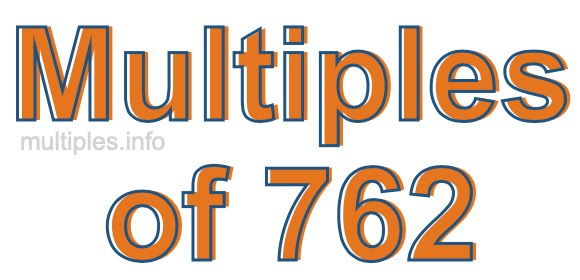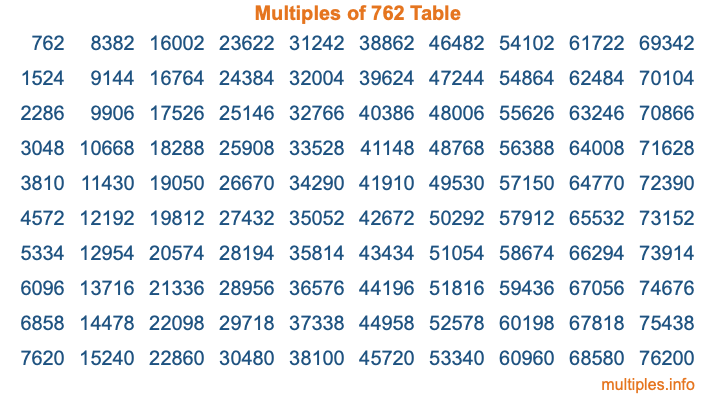Multiples of 762Welcome to the Multiples of 762 page. Here we will first teach you everything you will ever need to know about the multiples of 762, and then give you a study guide summary of everything we taught you to make sure you remember it all. Use this page to look up facts and learn information about the multiples of 762. This page will make you a multiples of seven hundred sixty-two expert!

Definition of Multiples of 762
Multiples of 762 are all the numbers that when divided by 762 equal an integer. Each of the multiples of 762 are called a multiple. A multiple of 762 is created by multiplying 762 by an integer.

Therefore, to create a list of multiples of 762, you start with 1 multiplied by 762, then 2 multiplied by 762, then 3 multiplied by 762, and so on for as long as you want. Thus, the list of the first five multiples of 762 is 762, 1524, 2286, 3048, and 3810. To see a larger list of multiples of 762, see the printable image of Multiples of 762 further down on this page. We also have a category where you can choose any nth multiple of 762.

Multiples of 762 Checker
The Multiples of 762 Checker below checks to see if any number of your choice is a multiple of 762. In other words, it checks to see if there is any number (integer) that when multiplied by 762 will equal your number. To do that, we divide your number by 762. If the the quotient is an integer, then your number is a multiple of 762.

Is  a multiple of 762?

Least Common Multiple of 762 and ...
A Least Common Multiple (LCM) is the lowest multiple that two or more numbers have in common. This is also called the smallest common multiple or lowest common multiple and is useful to know when you are adding our subtracting fractions. Enter one or more numbers below (762 is already entered) to find the LCM.

Check out our LCM Calculator if you need more details about the Least Common Multiple or if you need the LCM for different numbers for adding and subtraction fractions.

nth Multiple of 762
As we stated above, 762 is the first multiple of 762, 1524 is the second multiple of 762, 2286 is the third multiple of 762, and so on. Enter a number below to find the nth multiple of 762.

th multiple of 762

Multiples of 762 vs Factors of 762
762 is a multiple of 762 and a factor of 762, but that is where the similarities end. All postive multiples of 762 are 762 or greater than 762. All positive factors of 762 are 762 or less than 762.

Below is the beginning list of multiples of 762 and the factors of 762 so you can compare:

Multiples of 762: 762, 1524, 2286, 3048, 3810, etc.

Factors of 762: 1, 2, 3, 6, 127, 254, 381, 762

As you can see, the multiples of 762 are all the numbers that you can divide by 762 to get a whole number. The factors of 762, on the other hand, are all the whole numbers that you can multiply by another whole number to get 762.

It's also interesting to note that if a number (x) is a factor of 762, then 762 will also be a multiple of that number (x).

Multiples of 762 vs Divisors of 762
The divisors of 762 are all the integers that 762 can be divided by evenly. Below is a list of the divisors of 762.

Divisors of 762: 1, 2, 3, 6, 127, 254, 381, 762

The interesting thing to note here is that if you take any multiple of 762 and divide it by a divisor of 762, you will see that the quotient is an integer.

Multiples of 762 Table
Below is an image of the first 100 multiples of 762 in a table. The table is in chronological order, column by column. The first column has the first ten multiples of 762, the second column has the next ten multiples of 762, and so on.The Multiples of 762 Table is also referred to as the 762 Times Table or Times Table of 762. You are welcome to print out our table for your studies.

Negative Multiples of 762
Although not often discussed or needed in math, it is worth mentioning that you can make a list of negative multiples of 762 by multiplying 762 by -1, then by -2, then by -3, and so on, to get the following list of negative multiples of 762:

-762, -1524, -2286, -3048, -3810, etc.

Multiples of 762 Summary
Below is a summary of important Multiples of 762 facts that we have discussed on this page. To retain the knowledge on this page, we recommend that you read through the summary and explain to yourself or a study partner why they hold true.

There are an infinite number of multiples of 762.

A multiple of 762 divided by 762 will equal a whole number.

762 divided by a factor of 762 equals a divisor of 762.

The nth multiple of 762 is n times 762.

The largest factor of 762 is equal to the first positive multiple of 762.

762 is a multiple of every factor of 762.

762 is a multiple of 762.

A multiple of 762 divided by a divisor of 762 equals an integer.

762 divided by a divisor of 762 equals a factor of 762.

Any integer times 762 will equal a multiple of 762.

Multiples of a Number
Here you can get the multiples of another number, all with the same attention to detail as we did for multiples of 762 on this page.

Multiples of
Multiples of 763
Did you find our page about multiples of seven hundred sixty-two educational? Do you want more knowledge? Check out the multiples of the next number on our list!